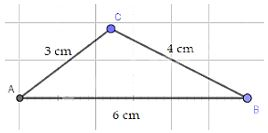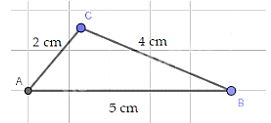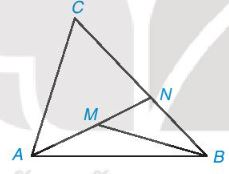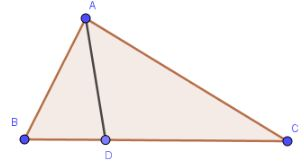## Solve Exercises Lesson 33 The relationship between the three sides of a triangle (Chapter 9 Math 7 Connection) – Math Book

Solve Exercises Lesson 33 The relationship between the three sides of a triangle (Chapter 9 Math 7 Connection)
============

Lesson 33 The relationship between the three sides of a triangle

### Solve lesson 9.10, page 69, Math 7 Textbook Connecting knowledge volume 2 – KNTT

Given sets of triplets of the following lengths:

a) 2 cm, 3 cm, 5 cm

b) 3 cm, 4 cm, 6 cm

c) 2 cm, 4 cm, 5 cm.

Which triple cannot be the lengths of the three sides of a triangle? Why? For each of the remaining triplets, draw a triangle with the lengths of the three sides given in that triple.

Detailed instructions for solving problems 9.10

Solution method

When checking if 3 line segments satisfy the triangle inequality, for the sake of brevity, we just need to check if the sum of the lengths of the 2 smaller sides is greater than the length of the largest side.

Detailed explanation

According to the triangle inequality:

a) Since 2 + 3 = 5, the set of three line segments of length 2 cm, 3 cm, and 5 cm cannot be the lengths of the three sides of a triangle.

b) Since 3+4 > 6, a set of three segments with lengths 3 cm, 4 cm, and 6 cm can be the lengths of the three sides of a triangle.* How to draw: + Draw the length of side AB = 6cm.

+ Using a compass, draw an arc with center A and radius 3 cm, and a circle with center B with a radius of 4 cm. These two arcs intersect at C.

We get the triangle ABC to draw.

c) Since 2+4 > 5, a set of three segments with lengths 2 cm, 4 cm, and 5 cm can be the lengths of the three sides of a triangle.* How to draw: + Draw the length of side AB = 5cm.

+ Using a compass, draw an arc with center A and radius 2 cm, and a circle with center B with a radius of 4 cm. These two arcs intersect at C.

We get the triangle ABC to draw.

–>

— *****

### Solve problem 9.11, page 69, Math 7 Textbook Connecting knowledge volume 2 – KNTT

a) Let ABC be a triangle with AB = 1 cm, BC = 7 cm. Find the length of the side CA knowing that it is an integer (cm).

b) Let ABC be a triangle with AB = 2 cm, BC = 6 cm and BC being the largest side. Find the length of the side CA knowing that it is an integer (cm).

Detailed instructions for solving problems 9.11

Solution method

Using triangle inequality: In a triangle, the length of one side is always less than the sum of the lengths of the other two sides and greater than the difference in the lengths of the other two sides: b – c < a < b + c (with a, b, c are the lengths of the three sides of the triangle)

Combine the condition that the side length CA is an integer

Detailed explanation

Applying the triangle inequality in triangle ABC, we have:

7 – 1 < CA < 7 + 1

6 < CA < 8

Where CA is an integer

CA = 7 cm.

So CA = 7 cm.

b) Applying the triangle inequality in triangle ABC, we have:

AB + CA > BC

2 + CA > 6

CA > 4 cm

Where CA is an integer and CA < 6 (because BC = 6 cm is the largest side of the triangle)

CA = 5 cm

So CA = 5 cm.

–>

— *****

### Solve lesson 9.12, page 69, Math 7 textbook Connecting knowledge volume 2 – KNTT

Let M lie inside triangle ABC. Let N be the intersection of line AM and side BC. (H.9.18)a) Compare MB with MN + NB, then infer MA + MB < NA + NB

b) Compare NA with CA + CN, then infer that NA + NB < CA + CB

c) Prove MA + MB < CA + CB.

Detailed instructions for solving problem 9.12

Solution method

Use theorem:

+ Among oblique lines and perpendicular lines drawn from a point outside a line to that line, the perpendicular is the shortest.

In a triangle, the side opposite the largest angle is the largest side.

Detailed explanation

a) 3 points M,N,B are not collinear.

Applying the triangle inequality in triangle MNB has:

MB < MN + NB

MA + MB < MA + MN + NB

MA + MB < NA + NB (because MA + MN = NA) (1)

b) 3 points A,N,C are not collinear.

Applying the triangle inequality in triangle ACN has:

NA < CA + CN

NA + NB < CA + CN + NB

NA + NB < CA + CB (because CN + NB = CB) (2)

c) From (1) and (2) we have:

MA + MB < NA + NB < CA + CB

So MA + MB < CA + CB

–>

— *****

### Solve problem 9.13, page 69, Math 7 Textbook Connecting knowledge volume 2 – KNTT

Given triangle ABC, point D lies between B and C. Prove that AD is less than half the perimeter of triangle ABC.

Detailed instructions for solving problem 9.13

Solution method

Apply the relationship between the three sides of triangle ABD and triangle ACD.

Detailed explanationApplying the relationship between the three sides of triangle ABD, we have: AD < AB + BD

Applying the relationship between the three sides of triangle ACD, we have: AD < CD + AC